Precalculus : Solve a Right Triangle

Example Questions

Example Question #1 : Solving Right Triangles

In a right triangle, if the hypotenuse isand a leg is, what is the area of the triangle?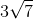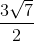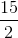Explanation:

Use the Pythagorean Theorem to find the other leg.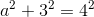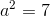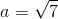The length of the given leg is 3, and the unknown leg is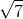.

Use the area of a triangle formula and solve.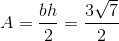Example Question #1 : Solving Right Triangles

An isosceles right triangle has a hypotenuse of 1.  What is the area of this triangle?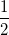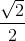Explanation:

Write the formula for the Pythagorean theorem.In an isosceles right triangle, both legs of the right triangle are equal.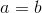Substitute the either variable and the known hypotheuse and determine the side length.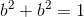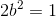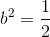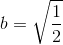This length represents both the base and the height of the triangle. Write the area of a triangle and substitute to solve for the area.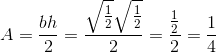Example Question #1 : Trigonometric Applications

Solve the right triangle.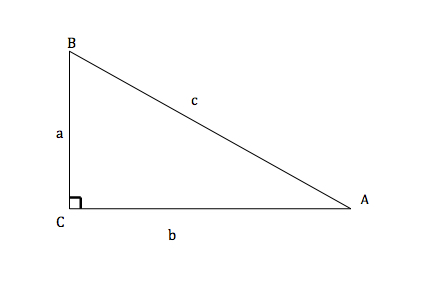Solve the right triangle given that a=5, b=12, and A=22.620°

B=67.380°

C=90°

c=17

B=67.380°

C=90°

c=13

B=90°

C=67.380°

c=13

None of these answers are correct.

B=90°

C=67.380°

c=169

B=67.380°

C=90°

c=13

Explanation:C is given as 90°.

A is given as 22.620°

a is given as 5

b is given as 12Therefore...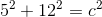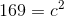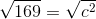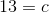All angles of a triangle add up to equal 180°.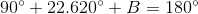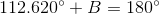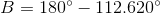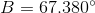Example Question #1 : Trigonometric Applications

Solve the right triangle.C=90°

B=45°

a=5

c=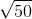A=45°

b=A=135°

b=5

A=135°

b=2.07

None of these answers are correct.

A=45°

b=5

A=45°

b=5

Explanation:Given that:

C=90°

B=45°

a=5

c=Therefore...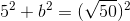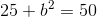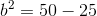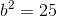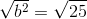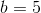All angles of a triangle add up to 180°.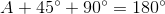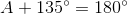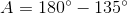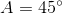Example Question #2 : Trigonometric Applications

A right triangle has a base of 10 and a hypotenuse of 20.  What is the length of the other leg?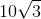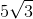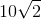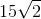Explanation:

Write the Pythagorean Theorem.Substitute the values of the leg and hypotenuse.  The hypotenuse is the longest side of the right triangle.  Solve for the unknown variable.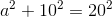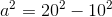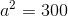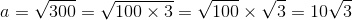Example Question #1 : Solve A Right Triangle

In the right triangle ABC, side AB iscm long, side AC iscm long, and side BC is the hypotenuse. How long is side BC?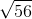cm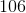cmcm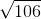cmcm
Given that ABC is a right triangle, the length of hypotenuse BC is the root of the sum of the squares of the two other sides (in other words,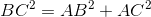. Since AB iscm long and AC iscm long, we get that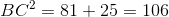, and so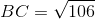.